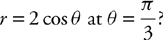# AP Calculus BC Practice Test 42

### Test Information10 questions20 minutes

Calculator Disallowed

1.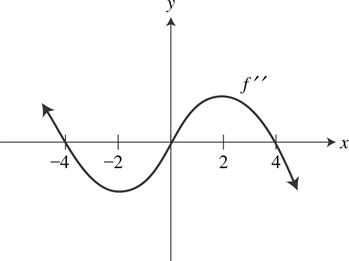The graph of f″, the second derivative of f is shown above. The graph of f″ has horizontal tangents at x =-2 and x = 2. For what values of x does the graph of the function f have a point of inflection?

2. Given the infinite series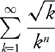, what are all values of n for which the series converges问号

3. If f(x) is continuous and f(x) > 0 on [0, 4] and twice differentiable on (0, 4) such that f′ (x) > 0 and f″(x) > 0 which of the following has the greatest value?

4. Which of the following is an equation of the tangent line to the curve described by the parametric equations x = t and y = t2 when t = 4 ?

5. Let f and g be differentiable functions such that g (x) = f-1 (x). If f(2) = 4, f(3) = 9,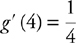and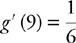, what is the value of f′ (3)?

6. What is the value of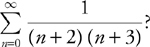7.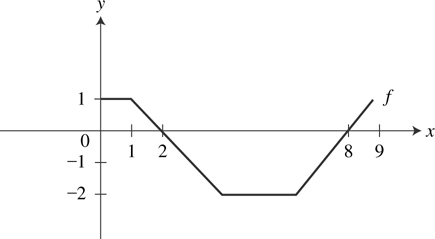The domain of the function f is 0 ≤ x ≤ 9 as shown above. If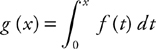, at what value of x is g (x) the absolute maximum?

8. Which of the following represents the arc length of the curve with the parametric equations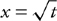and y = et for 4 ≤ t ≤ 9?

9. If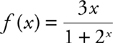the horizontal asymptotes of the graph of f is/are

10. Which of the following expressions gives the slope of the tangent line to the curve of the polar equation